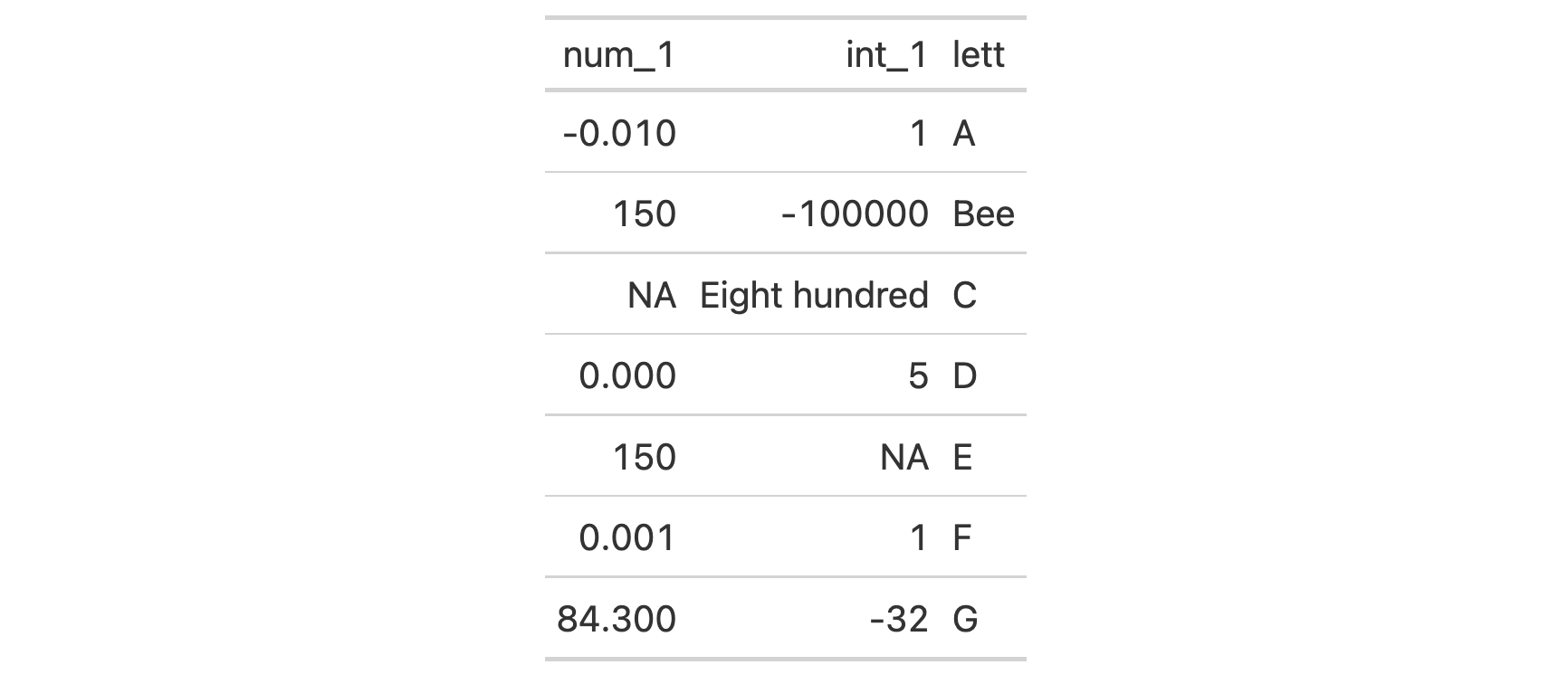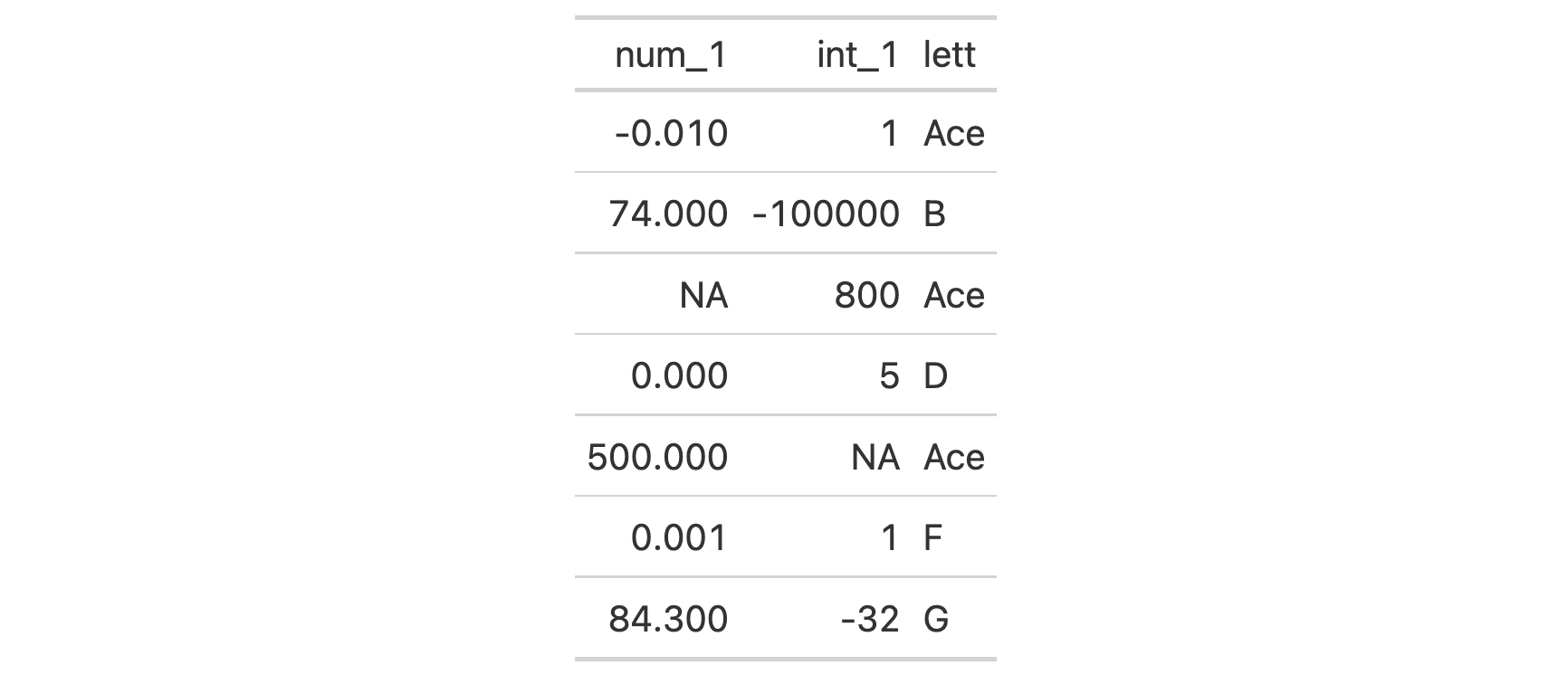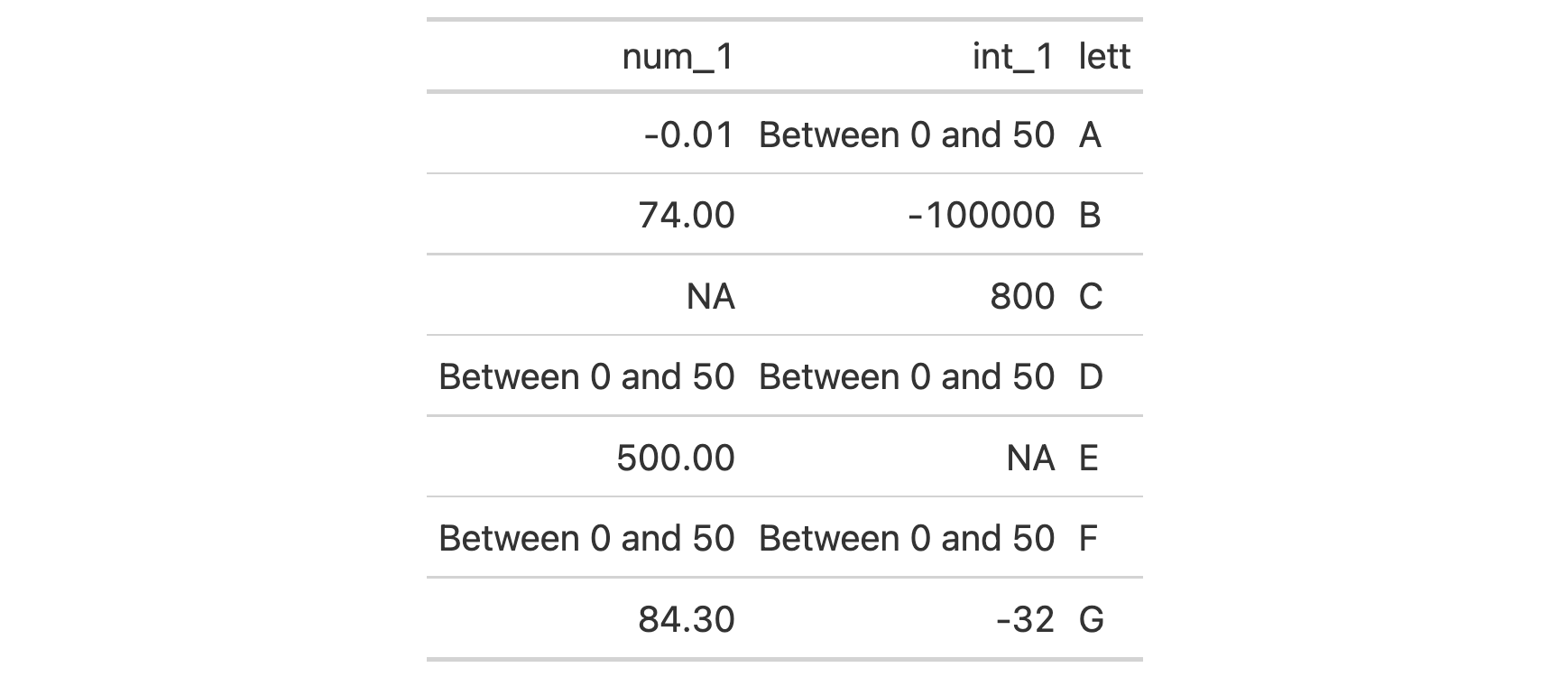Should you need to replace specific cell values with custom text, the sub_values() function can be good choice. We can target cells for replacement though value, regex, and custom matching rules.

## Usage

sub_values(
data,
columns = everything(),
rows = everything(),
values = NULL,
pattern = NULL,
fn = NULL,
replacement = NULL,
escape = TRUE
)

## Arguments

data

A table object that is created using the gt() function.

columns

Optional columns for constraining the targeting process. Providing everything() (the default) results in cells in all columns being targeting (this can be limited by rows however). Can either be a series of column names provided in c(), a vector of column indices, or a helper function focused on selections. The select helper functions are: starts_with(), ends_with(), contains(), matches(), one_of(), num_range(), and everything().

rows

Optional rows for constraining the targeting process. Providing everything() (the default) results in all rows in columns being targeted. Alternatively, we can supply a vector of row captions within c(), a vector of row indices, or a helper function focused on selections. The select helper functions are: starts_with(), ends_with(), contains(), matches(), one_of(), num_range(), and everything(). We can also use expressions to filter down to the rows we need (e.g., [colname_1] > 100 & [colname_2] < 50).

values

The specific value or values that should be replaced with a replacement value. If pattern is also supplied then values will be ignored.

pattern

A regex pattern that can target solely those values in character-based columns. If values is also supplied, pattern will take precedence.

fn

A supplied function that operates on x (the data in a column) and should return a logical vector that matches the length of x (i.e., number of rows in the input table). If either of values or pattern is also supplied, fn will take precedence.

replacement

The replacement value for any cell values matched by either values or pattern. Must be a character or numeric vector of length 1.

escape

An option to escape replacement text according to the final output format of the table. For example, if a LaTeX table is to be generated then LaTeX escaping would be performed on the replacements during rendering. By default this is set to TRUE but setting to FALSE would be useful in the case where replacement text is crafted for a specific output format in mind.

## Value

An object of class gt_tbl.

## Examples

Let's create an input table with three columns. This contains an assortment of values that could potentially undergo some substitution via sub_values().

tbl <-
dplyr::tibble(
num_1 = c(-0.01, 74, NA, 0, 500, 0.001, 84.3),
int_1 = c(1L, -100000L, 800L, 5L, NA, 1L, -32L),
lett = LETTERS[1:7]
)

tbl
#> # A tibble: 7 x 3
#>     num_1   int_1 lett
#>     <dbl>   <int> <chr>
#> 1  -0.01        1 A
#> 2  74     -100000 B
#> 3  NA         800 C
#> 4   0           5 D
#> 5 500          NA E
#> 6   0.001       1 F
#> 7  84.3       -32 G

Values in the table body cells can be replaced by specifying which values should be replaced (in values) and what the replacement value should be. It's okay to search for numerical or character values across all columns and the replacement value can also be of the numeric or character types.

tbl %>%
gt() %>%
sub_values(values = c(74, 500), replacement = 150) %>%
sub_values(values = "B", replacement = "Bee") %>%
sub_values(values = 800, replacement = "Eight hundred")We can also use the pattern argument to target cell values for replacement in character-based columns.

tbl %>%
gt() %>%
sub_values(pattern = "A|C|E", replacement = "Ace")For the most flexibility, it's best to use the fn argument. With that you need to ensure that the function you provide will return a logical vector when invoked on a column of cell values, taken as x (and, the length of that vector must match the length of x).

tbl %>%
gt() %>%
sub_values(
fn = function(x) x >= 0 & x < 50,
replacement = "Between 0 and 50"
)## Function ID

3-21

Other data formatting functions: data_color(), fmt_bytes(), fmt_currency(), fmt_datetime(), fmt_date(), fmt_duration(), fmt_engineering(), fmt_fraction(), fmt_integer(), fmt_markdown(), fmt_number(), fmt_partsper(), fmt_passthrough(), fmt_percent(), fmt_roman(), fmt_scientific(), fmt_time(), fmt(), sub_large_vals(), sub_missing(), sub_small_vals(), sub_zero(), text_transform()